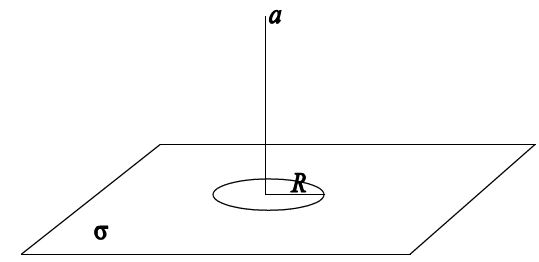# Find the Electric field!An infinitely large surface of uniform charge density $\sigma$ has a disc of radius $R$ cut out (see figure). The magnitude of the electric field at a distance $a$ above the center of the disc is given by?

×